Test: Probability- 1

# Test: Probability- 1

Test Description

## 10 Questions MCQ Test CSAT Preparation for UPSC CSE | Test: Probability- 1

Test: Probability- 1 for Quant 2023 is part of CSAT Preparation for UPSC CSE preparation. The Test: Probability- 1 questions and answers have been prepared according to the Quant exam syllabus.The Test: Probability- 1 MCQs are made for Quant 2023 Exam. Find important definitions, questions, notes, meanings, examples, exercises, MCQs and online tests for Test: Probability- 1 below.
Solutions of Test: Probability- 1 questions in English are available as part of our CSAT Preparation for UPSC CSE for Quant & Test: Probability- 1 solutions in Hindi for CSAT Preparation for UPSC CSE course. Download more important topics, notes, lectures and mock test series for Quant Exam by signing up for free. Attempt Test: Probability- 1 | 10 questions in 10 minutes | Mock test for Quant preparation | Free important questions MCQ to study CSAT Preparation for UPSC CSE for Quant Exam | Download free PDF with solutions
 1 Crore+ students have signed up on EduRev. Have you?
Test: Probability- 1 - Question 1

### A die is rolled twice. What is the probability of getting a sum equal to 9?

Detailed Solution for Test: Probability- 1 - Question 1

Total number of outcomes possible when a die is rolled = 6 (∵ any one face out of the 6 faces)

• Hence, total number of outcomes possible when a die is rolled twice, n(S) = 6 x 6 = 36

E = Getting a sum of 9 when the two dice fall = {(3,6), (4,5), (5,4), (6,3)}

• Hence, n(E) = 4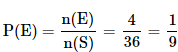Test: Probability- 1 - Question 2

### Hunar wrote two sections of CAT paper; Verbal and QA in the same order. The probability of her passing both sections is 0.6. The probability of her passing the verbal section is 0.8. What is the probability of her passing the QA section given that she has passed the Verbal section?

Detailed Solution for Test: Probability- 1 - Question 2
• Let P(QA) = passing QA’s section
• P(V) = passing Verbal section
• So, P(QA/V) = P(QA∩V)/P(V) = 0.6/0.8 = 0.75
Hence, the correct answer is option A.
Test: Probability- 1 - Question 3

### A randomly selected year is containing 53 Mondays then probability that it is a leap year

Detailed Solution for Test: Probability- 1 - Question 3

The correct option is A

• Selected year will be a non leap year with a probability 3/4
• Selected year will be a leap year with a probability 1/4
• A selected leap year will have 53 Mondays with probability 2/7
• A selected non leap year will have 53 Mondays with probability 1/7
• E→ Event that randomly selected year contains 53 Mondays

P(E) =  (3/4 × 1/7) + (1/4 × 2/7)
P(Leap Year/ E) = (2/28) / (5/28) = 2/5

Test: Probability- 1 - Question 4

One card is randomly drawn from a pack of 52 cards. What is the probability that the card drawn is a face card(Jack, Queen or King)

Detailed Solution for Test: Probability- 1 - Question 4
• Total number of cards, n(S) = 52
• Total number of face cards, n(E) = 12 (4 Jacks, 4 Queens, 4 Kings)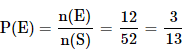Test: Probability- 1 - Question 5

A dice is thrown. What is the probability that the number shown in the dice is divisible by 3?

Detailed Solution for Test: Probability- 1 - Question 5
• Total number of outcomes possible when a die is rolled, n(S) = 6 (? 1 or 2 or 3 or 4 or 5 or 6)
• E = Event that the number shown in the dice is divisible by 3 = {3, 6}
Hence, n(E) = 2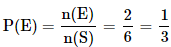Test: Probability- 1 - Question 6

John draws a card from a pack of cards. What is the probability that the card drawn is a card of black suit?

Detailed Solution for Test: Probability- 1 - Question 6

Total number of cards, n(S) = 52
Total number of black cards, n(E) = 26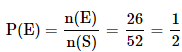Test: Probability- 1 - Question 7

Three coins are tossed. What is the probability of getting at most two tails?

Detailed Solution for Test: Probability- 1 - Question 7

Total number of outcomes possible when a coin is tossed = 2 (∵ Head or Tail)

• Hence, total number of outcomes possible when 3 coins are tossed, n(S) = 2 x 2 x 2 = 8
​(∵ S = {TTT, TTH, THT, HTT, THH, HTH, HHT, HHH})

E = event of getting at most two Tails = {TTH, THT, HTT, THH, HTH, HHT, HHH}

• Hence, n(E) = 7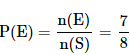Test: Probability- 1 - Question 8

A bag contains 4 black, 5 yellow and 6 green balls. Three balls are drawn at random from the bag. What is the probability that all of them are yellow?

Detailed Solution for Test: Probability- 1 - Question 8

Total number of balls = 4 + 5 + 6 = 15

Let S be the sample space.

• n(S) = Total number of ways of drawing 3 balls out of 15 = 15C3

Let E = Event of drawing 3 balls, all of them are yellow.

• n(E) = Number of ways of drawing 3 balls from the total 5 = 5C3
(∵ there are 5 yellow balls in the total balls)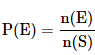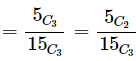[∵ nCr = nC(n-r). So 5C3 = 5C2. Applying this for the ease of calculation]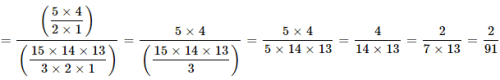Test: Probability- 1 - Question 9

A bag contains 2 yellow, 3 green and 2 blue balls. Two balls are drawn at random. What is the probability that none of the balls drawn is blue?

Detailed Solution for Test: Probability- 1 - Question 9

Total number of balls = 2 + 3 + 2 = 7

► Let S be the sample space.

• n(S) = Total number of ways of drawing 2 balls out of 7 = 7C2

► Let E = Event of drawing 2 balls, none of them is blue.

• n(E) = Number of ways of drawing 2 balls from the total 5 (= 7-2) balls = 5C2
(∵ There are two blue balls in the total 7 balls. Total number of non-blue balls = 7 - 2 = 5)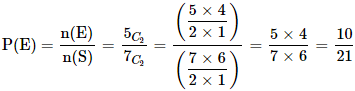Test: Probability- 1 - Question 10

There are 15 boys and 10 girls in a class. If three students are selected at random, what is the probability that 1 girl and 2 boys are selected?

Detailed Solution for Test: Probability- 1 - Question 10

Let S be the sample space.

• n(S) = Total number of ways of selecting 3 students from 25 students = 25C3

Let E = Event of selecting 1 girl and 2 boys

• n(E) = Number of ways of selecting 1 girl and 2 boys

15 boys and 10 girls are there in a class. We need to select 2 boys from 15 boys and 1 girl from 10 girls

Number of ways in which this can be done:
15C2 × 10C1
Hence n(E) = 15C2 × 10C1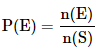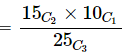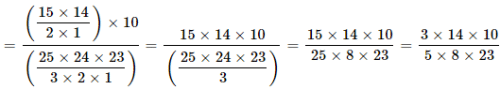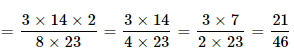## CSAT Preparation for UPSC CSE

72 videos|64 docs|92 tests
 Use Code STAYHOME200 and get INR 200 additional OFF Use Coupon Code
Information about Test: Probability- 1 Page
In this test you can find the Exam questions for Test: Probability- 1 solved & explained in the simplest way possible. Besides giving Questions and answers for Test: Probability- 1, EduRev gives you an ample number of Online tests for practice

## CSAT Preparation for UPSC CSE

72 videos|64 docs|92 tests